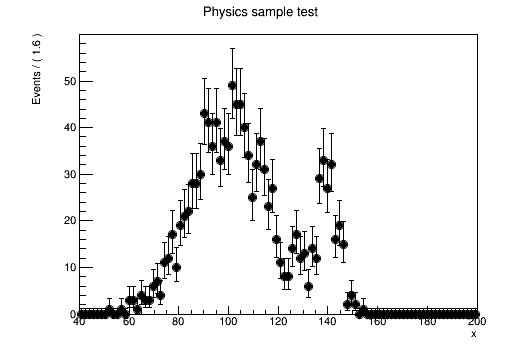# RooSimultaneous template fit

Dear experts,

I am currently trying to do a simultaneous fit in several variables/distributions in order to extract the number of events of given processes.
The first example  I came up with uses two distributions (in this example they are identical):
histograms_1 & histograms_2.
Further for each distribution I have the observed data, a signal template/pdf and a background template/pdf.
I use the latter to construct two models. So far everything works.

Now I would like to combine the two observed data samples:

```combined_data = deepcopy( roofit_histograms_1['data'] ) combined_data.add( roofit_histograms_2['data'] )```
where roofit_histograms_2[‘data’] is a RooDataHist.

Next I try to fit to this combined data:

which fails with

```[#0] ERROR:InputArguments -- RooTreeData::split(data) ERROR category sample doesn't depend on any variable in this dataset [#0] ERROR:Fitting -- RooAbsTestStatistic::initSimMode(nll_simPdf_data) ERROR: index category of simultaneous pdf is missing in dataset, aborting```

Obviously something is wrong with the way I create the combined data.
What is the correct way? How do I combine the two RooDataHist such that RooSimultaneous::fitTo is happy with it?

Luke

In a few examples I found on the internet, I also saw

```combined_data = RooDataHist('combined_data', 'combined_data', variables, RooFit.Index(sample), RooFit.Import('m1', roofit_histograms_1['data']), RooFit.Import('m2', roofit_histograms_2['data']))```
However, this way I do get a segmentation fault rather than the before-mentioned error.

Am I trying to do something unique? I am pretty sure that this is a very common use case.

I had a go at the RooFit example (rf501_simultaneouspdf) and tried to change it to my purposes.
The unchanged python translation can be found here:
gist.github.com/kreczko/682dc54 … eouspdf-py
It works fine.
Now, when I try to change the data input to a RooHistData, it does not work.
The example is here:
gist.github.com/kreczko/682dc54 … odified-py

The RooDataHist is created correctly while the fit does not work (all zeros)

The input histograms contains 1200 entries with the same mean and sigma (both background and signal are gaussians here). If I replace

``data = RooDataHist( 'real_data', 'real_data', RooArgList( x ), real_data )``

with

``data = model.generate( RooArgSet( x ), 1200 ) ``

It works.

I also tried to change the ‘comData’ (what is used as parameter for fitTo()) into a RooDataHist (using generated data), which works:

[quote]combData = RooDataSet( “combData”, “combined data”, RooArgSet( x ), RooFit.Index( sample ),
RooFit.Import( “physics”, data ),
RooFit.Import( “control”, data_ctl ) )
combData = combData.binnedClone() [/quote]

So something is going wrong in the combined RooDataSet creation when using RooDataHist instead of RooDataSet as input .

in fact, this is reproducable when changing the original example from

``RooDataSet combData("combData","combined data",x,Index(sample),Import("physics",*data),Import("control",*data_ctl)) ;``

to

``RooDataSet combData("combData","combined data",x,Index(sample),Import("physics",*data->binnedClone()),Import("control",*data_ctl)) ;``gist.github.com/kreczko/682dc542a9ae435ef630

The solution was to go via a map

```import rootpy.stl as stl MapStrRootPtr = stl.map(stl.string, "TH1*") StrHist = stl.pair(stl.string, "TH1*") real_data, real_data_ctl = get_data() input_hists = MapStrRootPtr() input_hists.insert(StrHist("physics", real_data)) input_hists.insert(StrHist("control", real_data_ctl )) combData = RooDataHist("combData", "combined data", RooArgList( x ), sample , input_hists)```

A big thanks to everyone that helped (Noel)!

Hello,
I see this is an old topic. I am trying to revive it, since I cannot get working the examples - none of the versions at https://gist.github.com/kreczko/682dc542a9ae435ef630 works for me.
My aim is to use python with RooSimultaneous with histograms RooDataHist.
Everytime, every example, root v6.16 and v6.18, rootpy or just pyroot plain - I end up with a similar error

``````NotImplementedError: pair<_Rb_tree_iterator<pair<const string,TH1*> >,bool> map<string,TH1*>::insert<std::pair<std::__cxx11::basic_string<char>, TH1 *> >(pair<string,TH1*>&& __x) =>
could not convert argument 1 (this method can not (yet) be called)
``````

Which always points to te problem of this object and its method input()

``````m1=ROOT.std.map('string, TH1*')()
#import rootpy.stl as stl  #rootpy version
#MapStrRootPtr = stl.map(stl.string, "TH1*") # rootpy version
``````

Is it due to my python installation? (Default ubuntu 1804 version : 3.6.8 , libs 3.6.7 )

C version works: Simultaneous RooDataHist (Map) combined with tutorials/rf501…C

Hello @jaromrax,

we support neither external github projects nor rootpy. I invite you to try
https://root.cern.ch/doc/master/rf501__simultaneouspdf_8py.html

with pyroot. Please note the difference between pyroot (officially supported) and rootpy (not supported).

If you run into problems with pyroot, let us know, but let’s not discuss in old posts.